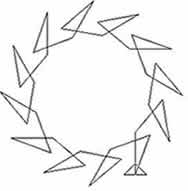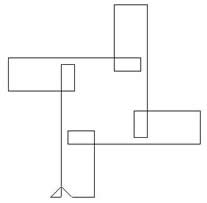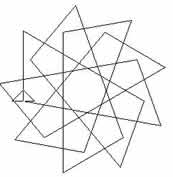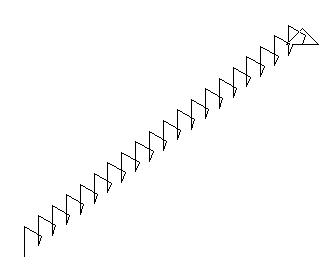#### You may also like### First Forward Into Logo 10: Count up - Count Down

What happens when a procedure calls itself?### LOGO Challenge - Pentagram Pylons

Pentagram Pylons - can you elegantly recreate them? Or, the European flag in LOGO - what poses the greater problem?### LOGO Challenge - Sequences and Pentagrams

Explore this how this program produces the sequences it does. What are you controlling when you change the values of the variables?

# Spiroflowers

##### Age 16 to 18Challenge Level

A spirolateral is a continuous path drawn by repeating a sequence of line segments of lengths $a_1, a_2, a_3, ... a_n$ with a given angle of turn between each line segment and the next one. (Alternatively the path can be considered as a repeated sequence of 'bound' vectors: $\overrightarrow {P_1P}_2, \overrightarrow{P_2P}_3,... \overrightarrow{P_n P}_{n+1}$, each vector starting at the endpoint of the previous vector.)In the first diagram the lengths of the line segments are equal and the angles of turn vary periodically in sequences of length 3. In the second diagram the lengths of the line segments vary periodically in sequences of length 5 and the angles of turn are equal. In the third diagram both the lengths and the angles vary.

Investigate these patterns, give sequences of instructions which would produce similar paths and explain why in each case the spirolateral paths are closed producing a cyclic pattern when the sequence is repeated infinitely often.Why does the spirolateral in this diagram continue indefinitely, shooting off to infinity if the sequence is repeated infinitely often?